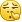﻿ 雨林寒舍 | CSS3+JS+PHP制作实时时钟
• 展开微博窗口
• QQ:52619941
• 微信:cnmemory
• 展开分类目录
• 还没有账号？

# CSS3+JS+PHP制作实时时钟

``````date_default_timezone_set('Asia/Shanghai');
\$sec = date('s');
\$min = date('i')+\$sec/60;
\$hour = date('H')+\$min/60;
\$hour_deg = floor(\$hour/12*360);
\$min_deg = floor(\$min/60*360);
\$sec_deg = floor(\$sec/60*360);``````

``````@keyframes sec_rotate {
from {transform: rotate(<?=(\$sec_deg)?>deg);} to {transform: rotate(<?=(360+\$sec_deg)?>deg);}
}``````

`animation: sec_rotate 60s infinite linear;`

``````<span class="digit">1</span>
<span class="digit">2</span>
<span class="digit">3</span>
<span class="digit">4</span>
<span class="digit">5</span>
<span class="digit">6</span>
<span class="digit">7</span>
<span class="digit">8</span>
<span class="digit">9</span>
<span class="digit">10</span>
<span class="digit">11</span>
<span class="digit">12</span>``````

``````\$(document).find('.digit').each(function(i){
var deg = (i + 1) * 30, rad = (Math.PI/180)*(deg-90);
var x = Math.round( Math.cos(rad) * 110 ), y = Math.round( Math.sin(rad) * 110 );
this.style.cssText='-webkit-transform: translate3d('+x+'px,'+y+'px,0px);-moz-transform: translateX('+x+') translateY('+y+');transform: translate3d('+x+'px,'+y+'px,0)';
});``````

# 评论

1.凌薇 #1

楼上的稍等啦

回复
2015-12-2
2.#2

打算撒是啊

回复
2015-11-30
3.n年那时 #3

博主，发表文章时，COPE部分代码区域的背景还有样式是怎样弄的呢

回复
2015-01-2
4.寻寻觅博客 #4

博主 我下载之后安装失败是什么原因啊

回复
2014-09-11
••life

用ftp放上去也还是不显示

回复
2014-10-19
5.寻寻觅博客 #5

感觉你真厉害啊

回复
2014-09-11
6.灰常记忆 #6不想折腾代码了

回复
2014-08-25
7.Memory #7

测试一下原生评论

回复
2014-08-22
8.呵呵 #8

牛逼

回复
2014-08-22